Technical Information Site of Power Supply Design

2017.06.08 Transfer Function

# Switching Transfer Functions: Derivation of Step-down Mode Transfer Functions Serving as a Foundation

DC/DC Converters: Sharing of Transfer Functions among Control Systems

In this chapter, and keeping in mind the equations that have been derived thus far, we first derive step-down mode switching transfer functions that will become a foundation for further analysis, and then derive unified transfer functions that use a state-space averaging method and do not specify an operating mode.

We will be blunt in stating clearly that these are probably the transfer functions that are hardest to visualize. For this reason, we begin by summarizing the flow of this chapter in Fig. 1.

Well then, upon hearing about a "state-space averaging method", many readers may feel somewhat confused. However, if this can be used, transfer functions for different converters can be derived according to an integrated approach. We will explain things so as to be easily understood. In any case, we hope you will make an effort to understand this section.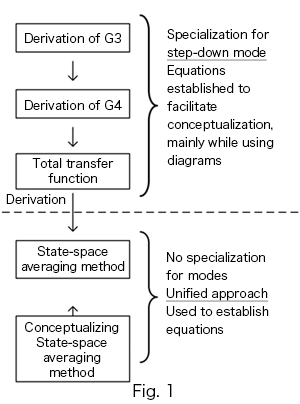G3Transfer Function Derivation, Frequency Characteristic
Initially, it is necessary to recall a switching regulator, broken down into function blocks. Here we shall derive transfer functions relating to the switching block G3.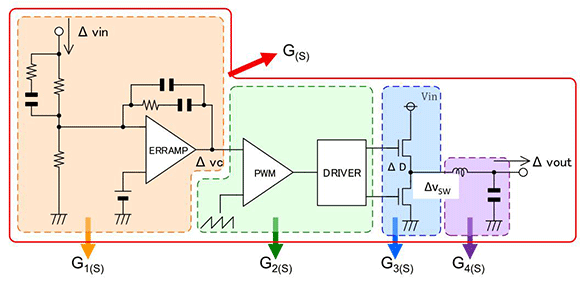First, we determine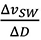Please look at Fig. 2.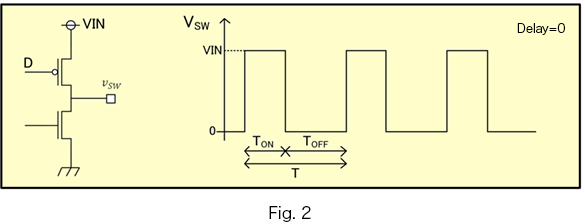This is a step-down mode switching waveform. VSW can be expressed in terms of the time average of H(VIN)) of the SW pin and L(O), and therefore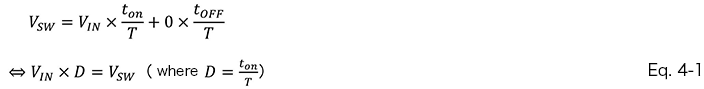If in equation 4-1D→D+∆D、VSW→VSW+∆VSW, then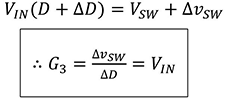can be derived.

This result indicates that G3 is such that the gain is constant and the phase does not change, as indicated in the diagram on the right.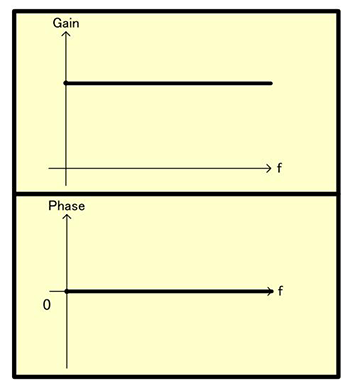Derivation of the Transfer Function G4

Next, G4, the output filter block,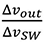is derived. Let's look at Fig. 4.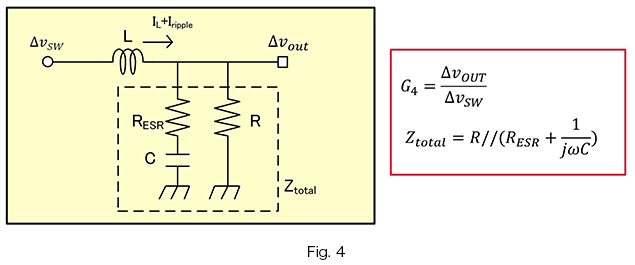This shows the path from VSW through the LC filter leading to Vout. Seen from VSW, Vout can be considered to be the impedance fraction of L and Ztotal, and so we can derive equation 4-2 below.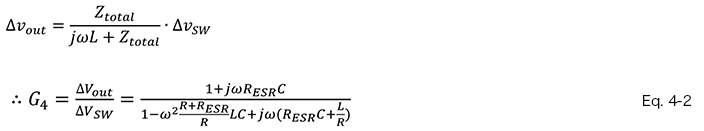Based on the transfer function for G4, we consider two cases.

Case 1

If it happens that RESR=0, R=open(∞), then G4 is as follows.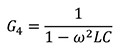This is a well-known LC filter, the Bode diagram for which is on the right. The reader has perhaps already grasped this much.

When f is small, G4 has a positive (>0) value, and so the phase is always 0.

When f becomes larger, increasing to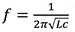, then the denominator becomes 0, and so the gain diverges.

As f further increases, G4 becomes a negative (<0) value, and so the phase rotates 180°.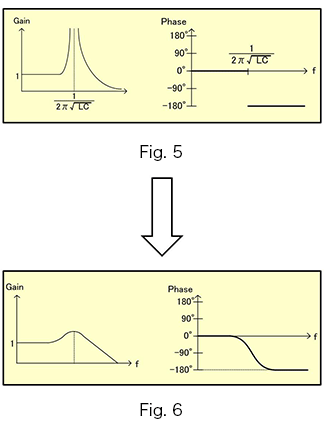Case 2

In Case 1, when RESR and R were added, a first-order imaginary term was added to the denominator. When f is sufficiently small, or when f is sufficiently large, this is the same as Case 1; but when f is near a resonance point, the denominator cannot become 0, and so the gain doesn’t diverge.

Further, here a first-order imaginary term remains, and so the phase does not go from 0 to 180°, but rather changes from 0 to 90° to 180°. Figure 6 is a Bode diagram showing this.

Hopefully the reader has grasped the relationship between the G4 transfer function and the Bode diagram.

Total Transfer Functions (general voltage step-down type)

From here, we use the transfer functions derived thus far to determine the total transfer function for the entire system. It was previously explained that the total transfer function can be represented by the following equation.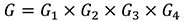Based on this, G can be expressed as follows.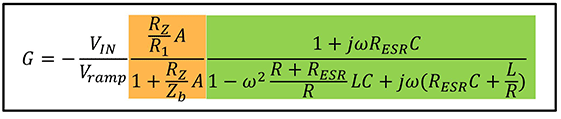The Bode diagram for this equation is as shown in Fig. 7. The features of each block can be seen. The color coding of the equations and the rectangles surrounding regions in the graph correspond.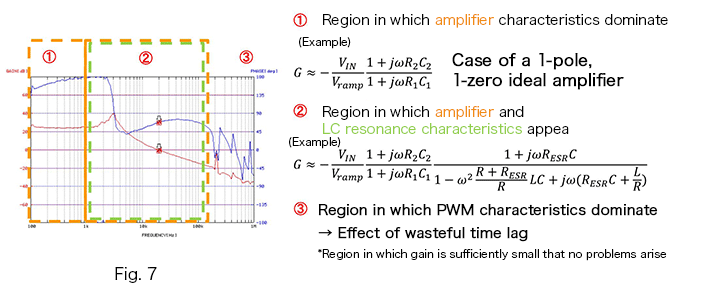In this way, if transfer functions can be represented in formula form for each block, the Bode diagram for the system can be drawn. However, in the discussion above the details were kept simple to facilitate understanding, and some parts of the presentation were not rigorous. Where G3 and G4 were derived, this approach can be applied for voltage step-down mode, but not in step-up or buck/boost modes. It is necessary to introduce a state-averaged approach that can be applied to all these modes.